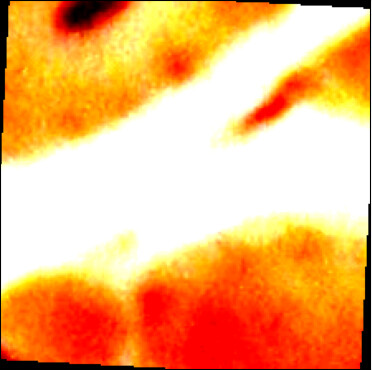# Not clear about the sequence of transforms.Normalize and transforms.ToTensor

Working with RGB image and binary mask as a target, I am confused about transformations.

Is it necessary to rescale the image and target between [0, 1] before feeding to the network? If so, is there any preference between `transforms.ToTensor` or `F.to_tensor`?

Is it also necessary to normalize the RGB images? If yes, I have the following working:

``````img_transform = transforms.Compose([
transforms.ToPILImage(),
transforms.RandomVerticalFlip(),
transforms.RandomHorizontalFlip(),
transforms.RandomCrop(size=(patch_size, patch_size), pad_if_needed=True), #these need to be in a reproducible order, first affine transforms and then color
transforms.RandomResizedCrop(size=patch_size),
transforms.RandomRotation(180),
transforms.ToTensor(),
transforms.Normalize(mean=[0.485, 0.456, 0.406],
std=[0.229, 0.224, 0.225]),
])
``````

But after applying the transformation the data from the loader is not between [0, 1] rather some other value like [+2.6, -2.11].
Do we rescale them again between [0, 1]? Then how to do that as `transforms.ToTensor` accepts only tensors.

So the output from `DataLoader` with Normalization:removing the Normalization:You don’t need to normalize the inputs, but it usually help training the model.
`torchvision.transforms.ToTensor()` and `torchvision.transforms.functional.to_tensor()` are applying the same transformation via an object or the functional API (`ToTensor` calls internally into `to_tensor` as seen here).

`Normalize` subtracts the mean and divides by the stddev to create an output with zero mean and unit variance, so the observed values would be expected.

Thanks for replying, so if we use `Normalize` are we working with data range beyond [0, 1]? And that’s completely ok for a network to function properly?

Yes, the range could be outside of `[0, 1]` and would ideally have a zero mean and unit variance.
Also yes, that’s beneficial for the network training. ML literature calls this normalization often “whitening” and you should be able to find more explanations e.g. in Bishop’s Pattern Recognition and Machine Learning or other references.

1 Like